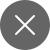# 13 Ultimate Seaborn tricks using Python

2+

Seaborn, another influential Python library used for the visualization of the data. Seaborn gives good insights into the data & gives us attractive statistical graphs, which provide a very high-level interface, much suitable while presenting the EDA(Exploratory Data Analysis) & preprocessing of the data to the client.

Seaborn graphs can be used to plot different graphs like the boxplot graph, barplot graphs, point plot graphs, etc. It can also be used to display univariate distribution & bivariate distribution, to check skewness, etc.

In this tricks & tutorial article, we will be showing step by step codes in Python, always remember, performing EDA & Data preprocessing is a must before applying your Machine Learning codes as it is a post mortem of the data for Data Scientists.

Firstly, we will start by importing the basic libraries of Python.

Then we will read the data using pd.read_csv and get an overview of the data using the head function. We are taking a simple dataset of Automobile.csv, the dataset can be found on most platforms, as it is the basic dataset.

Now plotting univariate distributions using distplot() function, the most convenient function for univariate distribution.

In seaborn, it is very much possible to visualize two variables and their relationship. So, plotting bivariate distribution using a jointplot() function.

We can also make hex bin plots using a jointplot() function. Hex bin plots break the two-dimensional area into hexagons.

We can plot multiple pairwise relationships of multiple variables of the data set using a pairplot() function.

There is a function in seaborn, a stripplot(), however, the scatter points usually overlap, to adjust their positions we can use ‘jitter=True’.

Using swarmplot() function. It positions each scatterplot point on a categorical axis, thereby avoiding overlapping points.

Using boxplot() function. The boxplots distribute the data in such a way that summarises a 5-point summary statistic of the data. A 5-point summary statistic of the data includes the minimum, maximum, 1st Quartile, 2nd Quartile, and the 3rd Quartile. The dots shown in the diagram below shows the number of outliers present in the dataset.

Similarly, we can use barplot() function, countplot() function, and pointplot() function to display a number of observations of a dataset.

Using seaborn we can draw multiple categories based on the categorical plot using catplot() function.

Finally, the ultimate hack is to quickly plot a linear regression model using seaborn.

This concludes our data visualization segment using Seaborn. If you wish to know more about sea born using python visit this https://seaborn.pydata.org/ to have a more advance look into data visualization using Python.

For more tutorials on Data Science/Machine Learning using Python, click few of the links below:2+

### 2 Responses

1. October 15, 2020

[…] Seaborn: pip install seaborn […]

0
2. December 2, 2020

[…] Data visualization and interpretation […]

0